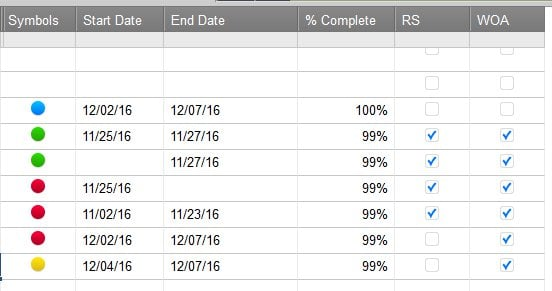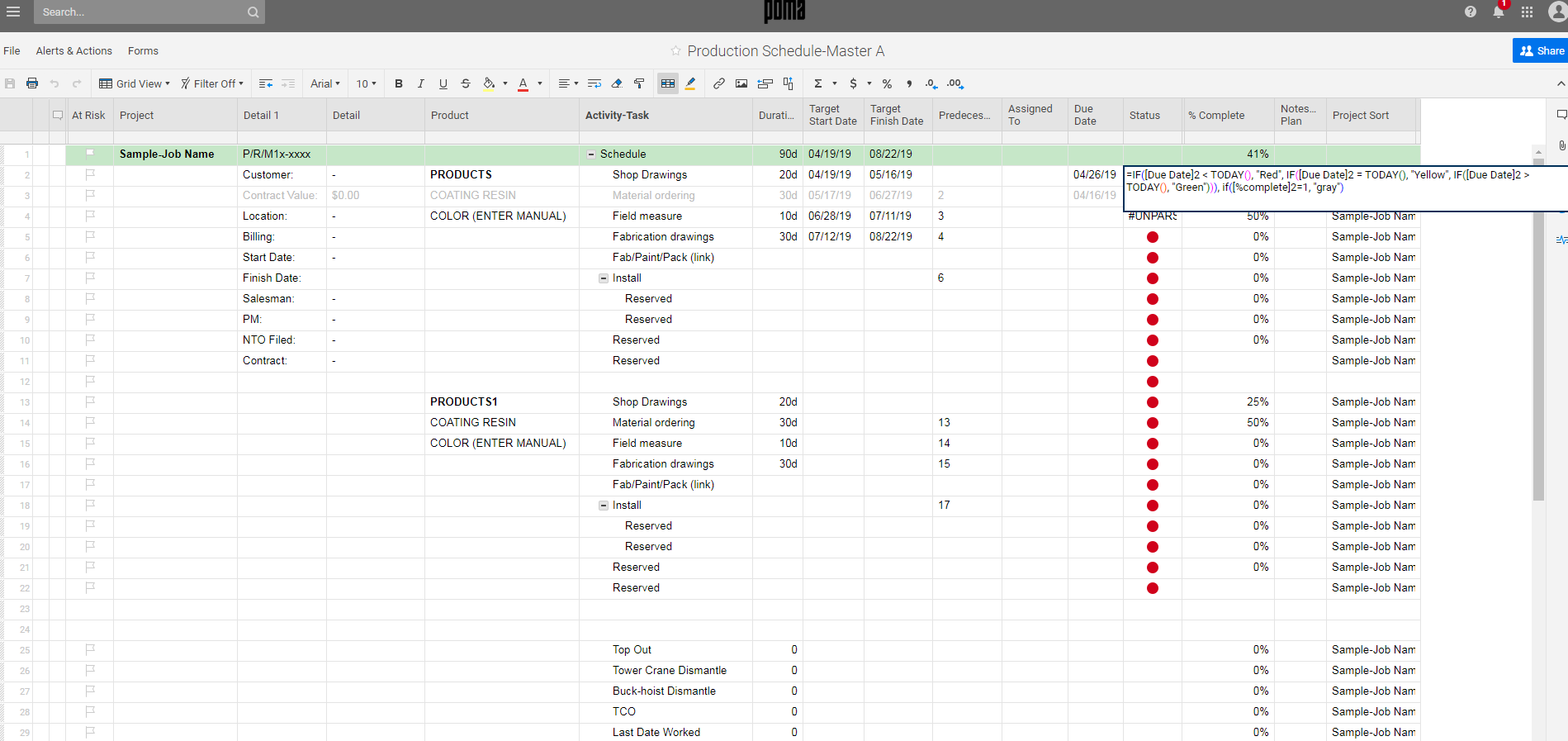## Automating RYG balls based on several columns

#### Welcome to the Smartsheet Forum Archives

The posts in this forum are no longer monitored for accuracy and their content may no longer be current. If there's a discussion here that interests you and you'd like to find (or create) a more current version, please Visit the Current Forums.

# Automating RYG balls based on several columns

I am trying to automate the RYG ball status column, based on several factors.

Red

- if not marked 100% complete in the [% Complete] column after the [End Date] or;

- if less than 7 days before [Start Date] and [RS] and [WOA] are not both =1

Yellow

- If greater than 7 days before [Start Date], [RS] and [WOA] are not both =1

Green

- If today is before [End Date] and [RS] and [WOA] are both =1

Blue

- If [% Complete] = 100%

[% Complete] is formatted for %

[Start Date} and [End Date] are of course, formatted as dates.

[RS] and [WOA] are both check boxes.

I end up getting turned around in nested IF statements and am ready to pull my hair out...

Tagged:

• Derrick,

This might work:

=IF([% Complete]13 = 1, "Blue", IF(AND(RS13, WOA13), IF([End Date]13 > TODAY(), "Green", "Red"), IF([Start Date]13 - 7 < TODAY(), "Red", "Yellow")))

Here's a screen shot: of the results.

Today is 11/26/16

Hope this helps.

Craig• I've used a similar formula in my spreadsheet to automate the RYG balls.   But what I've noticed is that it doesn't always work.  Often I have to go into the sheet and copy paste the formula from previous lines as users add more lines to the sheet because the formula does not copy itself.

Do you know what the reasons are this could happen?  Is it the way the users are adding information?

• Sarah,

Auto-fill looks at the 2 rows above or below the new row to determine if there should be formatting or formulas in the new row.

I've seen instances where formating only needs one row above.

How are the users adding rows?

If someone overwrites the formula, it is gone from the cell and can mess up later row additions.

The formula I gave also does not take into many 'edge' cases but should cover most of the common combinations.

Hope this helps.

Craig

• That was extremely helpful Craig.  Pretty much nailed it.  Thank you very much!

Derrick

• Need help automating RYG status with 2 columns:

If End Date is in the past, and % Complete is less than 100%, RED.

If End Date is 4 days away, and % Complete is less than 70%, YELLOW.

If End Date is 6+ days away, and % Complete is more than 50%, GREEN.

Thank you,

• ActivateDavid,

You have many missing conditions, even assuming you mean 4 days away OR LESS.

End Date is tomorrow and % Complete is 71%, for example.

https://www.smartsheet.com/blog/support-tip-build-nested-IF?_ga=2.142029499.84749476.1507748042-951982875.1506654632

Craig

• Need some insight on how to automate RYGG balls. I was successful in the first portion of the formula but want to add an additional conditional formula so that if the % complete column equals 100% then the ball will turn gray. See snapshot.

=IF([Due Date]2 < TODAY(), "Red", IF([Due Date]2 = TODAY(), "Yellow", IF([Due Date]2 > TODAY(), "Green")))